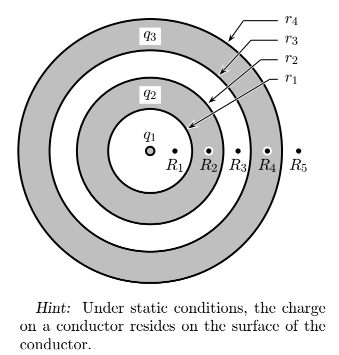# Problem: A point charge q1 is concentric with two spherical conducting thick shells, as shown in the figure below. The smaller spherical conducting shell has a net charge of q2 and the larger spherical conducting shell has a net charge of q3.  What is the charge Q r3 on the inner surface of the larger spherical conducting shell?1. Qr3 = −q1 − q2 + q32. Qr3 = +q13. Qr3 = +q1 − q24. Qr3 = −q15. Qr3 = −q1 − q2 − q36. Qr3 = +q1 + q27. Qr3 = 08. Qr3 = −q1 − q29. Qr3 = +q1 + q2 + q310. Qr3 = −q1 + q2

###### Problem Details

A point charge q1 is concentric with two spherical conducting thick shells, as shown in the figure below. The smaller spherical conducting shell has a net charge of q2 and the larger spherical conducting shell has a net charge of q3.  What is the charge Q r3 on the inner surface of the larger spherical conducting shell?

1. Qr3 = −q1 − q2 + q3

2. Qr3 = +q1

3. Qr3 = +q1 − q2

4. Qr3 = −q1

5. Qr3 = −q1 − q2 − q3

6. Qr3 = +q1 + q2

7. Qr3 = 0

8. Qr3 = −q1 − q2

9. Qr3 = +q1 + q2 + q3

10. Qr3 = −q1 + q2